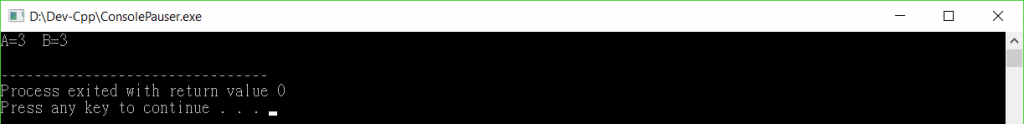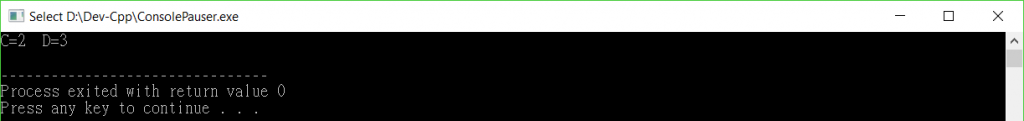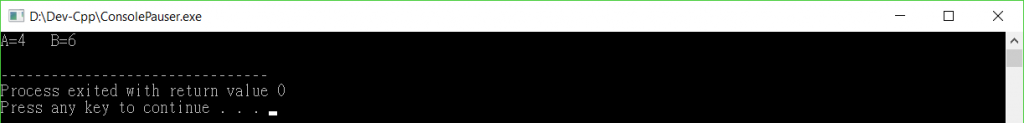#2018 iT 邦幫忙鐵人賽DAY 6
1
Software Development

## i++ 與 ++i可以怎麼理解？

``````int main(){
int A=2;
int B=2;
A++;
++B;
printf("A=%d  B=%d \n",A,B);
return 0;
}
````````````int main(){
int A = 2;
int B = 2;
int C = A++;
int D = ++B;
printf("C=%d  D=%d \n",C,D);
return 0;
}
``````A++：意思是先把A放到C，再執行A=A+1 的動作。

++B：意思是先再執行B=B+1，再把B放到D。

＝＝＝＝＝＝＝＝＝＝＝＝＝分格線＝＝＝＝＝＝＝＝＝＝＝＝＝

``````int main(){
int A=2 , AA=0;
int B=2 , BB=0;

AA = (A++) + (A++);
BB = (++B) + (B++);

printf("A=%d   B=%d\n",AA,BB);
return 0;
}
``````

`AA = (A++) + (A++);`

(A++) + (A++) ： 左方的A++右方的A++，也均為2，所以我們可以理解為AA=2+2，所以AA值為4
＝＝＝＝＝＝＝＝＝＝＝＝＝

`BB = (++B) + (B++);`

(++B) + (B++) ： 左方的++B右方的B++，也均為3，所以我們可以理解為BB=3+3，所以BB值為6＝＝＝＝＝＝＝＝＝＝＝＝＝分格線＝＝＝＝＝＝＝＝＝＝＝＝＝

``````int A=0;   //XXXXXX
int B=0;   //XXXXXX
int C=0;   //XXXXXX
``````